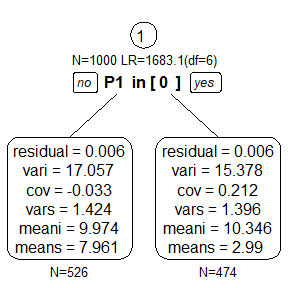# Getting Started with the semtree package

We first load the semtree package and the OpenMx package for specifying our SEM.

library(semtree)
library(OpenMx)

## Simulate data

Now, we simulate some data from a linear latent growth curve model (that is, a random intercept and random slope over time). The dataset will be called growth.data. The dataset contains five observations for each individual (X1 to X5) and one predictor P1. The predictor is dichotomous and predicts a (quite large) difference in mean slope.

set.seed(23)
N <- 1000
M <- 5
icept <- rnorm(N, 10, sd = 4)
slope <- rnorm(N, 3, sd = 1.2)
p1 <- sample(c(0, 1), size = N, replace = TRUE)
x <-
matrix(rep(icept, each = M), byrow = TRUE, ncol = M) +
rnorm(N * M, sd = .08)
growth.data <- data.frame(x, factor(p1))
names(growth.data) <- c(paste0("X", 1:M), "P1")

## Specify an OpenMx model

Now, we specify a linear latent growth curve model using OpenMx’s path specification. The model has five observed variables. Residual variances are assumed to be identical over time.

manifests <- names(growth.data)[1:5]
growthCurveModel <- mxModel("Linear Growth Curve Model Path Specification",
type="RAM",
manifestVars=manifests,
latentVars=c("intercept","slope"),
mxData(growth.data, type="raw"),
# residual variances
mxPath(
from=manifests,
arrows=2,
free=TRUE,
values = c(.1, .1, .1, .1, .1),
labels=c("residual","residual","residual","residual","residual")
),
# latent variances and covariance
mxPath(
from=c("intercept","slope"),
arrows=2,
connect="unique.pairs",
free=TRUE,
values=c(2, 0, 1),
labels=c("vari", "cov", "vars")
),
mxPath(
from="intercept",
to=manifests,
arrows=1,
free=FALSE,
values=c(1, 1, 1, 1, 1)
),
mxPath(
from="slope",
to=manifests,
arrows=1,
free=FALSE,
values=c(0, 1, 2, 3, 4)
),
# manifest means
mxPath(
from="one",
to=manifests,
arrows=1,
free=FALSE,
values=c(0, 0, 0, 0, 0)
),
# latent means
mxPath(
from="one",
to=c("intercept", "slope"),
arrows=1,
free=TRUE,
values=c(1, 1),
labels=c("meani", "means")
)
) # close model

# fit the model to the entire dataset
growthCurveModel <- mxRun(growthCurveModel)
#> Running Linear Growth Curve Model Path Specification with 6 parameters

## Run a tree

Now, we grow a SEM tree using the semtree function, which takes the model and the dataset as input. If not specified otherwise, SEM tree will assume that all variables in the dataset, which are not observed variables in the dataset are potential predictors.

tree <- semtree(model = growthCurveModel,
data = growth.data)

## Plotting

Once the tree is grown, we can plot it:

plot(tree)